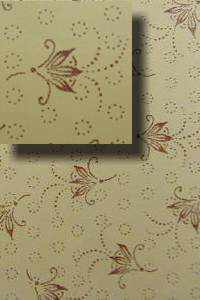# Crack and Peel Trunk Liner in Red and Tan T-172

Regular price
\$1.72
Sale price
\$1.72
Unit price
per

T-172 is interior trunk lining with a cream background and burgundy print.  The paper is 9 1/2" wide and is sold by the running foot or in a continuous roll. A crack and peel backing make this paper easy to apply to the interior of your trunk. To calculate how many feet of the paper liner you will need, you can following the following steps: (1)  Calculate the square inches sides, top and bottom by measure the length and width (remember that trunks have two short and two long sides.)  For example, a trunk is 36 inches long by 18 inches wide and is 20 inches tall. The short sides are 18" by 20" and there are two of them, so it would be 18 X 20 X 2 =  720 square inches. The sides are 36" long by 20" tall and there are two of them as well so it would be 36 X 18 X 2 = 1,296 square inches The bottom is 36" by 18" so it would be 36 X 18 = 648 square inches The total square inches for the bottom portion of the trunk is 720 + 1,296 + 648 = 2,664 square inches Repeat the process for the lid of the trunk and any insert you want to cover and add up all of your totals. Please note that if you have a domed, lid, remember to calculate the height of the lid using the highest point so ensure proper coverage.   (2)  The paper is sold by the running foot and is 9" wide so is 108" square inches per foot.   To determine the number of running feet you will need, take the total number of square inches you calculated for your trunk and divide it by 108.  In the example, the bottom of this trunk would be 2,664/108 = 24.667 running feet so would round up to the next whole number of 25 feet of trunk liner.    (3)  To be sure you have enough paper to match seems and have a bit of extra for the unexpected, were recommend adding an additional 10% or in this case,m three extra feet. If after you remove the paper liner there is still an odor, you can place a few charcoal bricks or a bowl of baking soda in the base of the trunk and close it up for a few days.  Either method should help to absorb some of the odor.  If make take a few tries to get the results you are looking for.